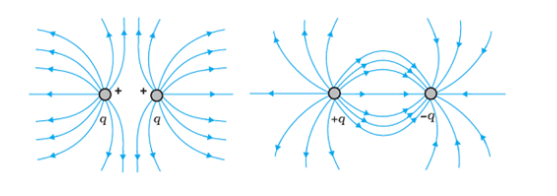# ﻿The electric field at a point is

Question:

The electric field at a point is

(a) always continuous

(b) continuous if there is no charge at that point

(c) discontinuous only if there is a negative charge at that point

(d) discontinuous if there is a charge at that point

Solution:

As we know, electric field lines emanate from positive charges and terminate on negative charges. Thus, electric field due to the charge Q will be continuous, if there is no charge at that point. It will be discontinuous if there is a charge at that point.

This can be understood with the help of figures shown below